# Notes on solving PDEs by the Finite Element Method¶

The Finite Element Method (FEM) is the numerical method for solving Partial Differential Equations (PDEs). FEM was developed in the middle of XX. century and now it is widely used in different areas of science and engineering, including mechanical and structural design, biomedicine, electrical and power design, fluid dynamics and other. FEM is based on a very elegant mathematical theory of weak solution of PDEs. In this section we will briefly discuss basic ideas underlying FEM.

## Strong form of Poisson’s equation and its integration¶

Let us start our discussion about FEM with the strong form of Poisson’s equation

(1)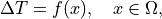(2)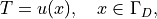(3)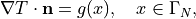where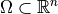is the solution domain with the boundary,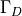is the part of the boundary where Dirichlet boundary conditions are given,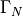is the part of the boundary where Neumann boundary conditions are given,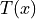is the unknown function to be found,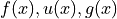are known functions.

FEM is based on a weak formulation. The weak form of the equation (1) is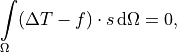whereis a test function. Integrating this equation by parts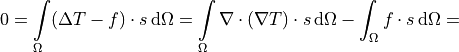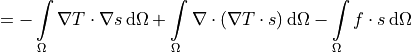and applying Gauss theorem we obtain: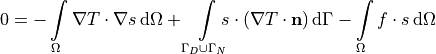or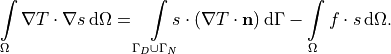The surface integral term can be split into two integrals, one over the Dirichlet part of the surface and second over the Neumann part

(4)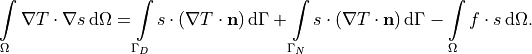The equation (4) is the initial weak form of the Poisson’s problem (1)(3). But we can not work with it without applying the boundary conditions. So it is time to talk about the boundary conditions.

### Dirichlet Boundary Conditions¶

On the Dirichlet part of the surface we have two restrictions. One is the Dirichlet boundary conditions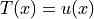as they are, and the second is the integral term overin equation (4). To be consistent we have to use only the Dirichlet conditions and avoid the integral term. To implement this we can take the function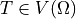and the test function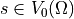, where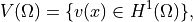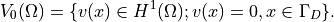In other words the unknown function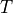must be continuous together with its gradient in the domain. In contrast the test functionmust be also continuous together with its gradient in the domain but it should be zero on the surface.

With this requirement the integral term over Dirichlet part of the surface is vanishing and the weak form of the Poisson equation forandbecomes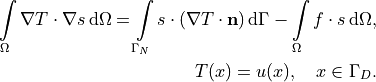That is why Dirichlet conditions in FEM terminology are called Essential Boundary Conditions. These conditions are not a part of the weak form and they are used as they are.

### Neumann Boundary Conditions¶

The Neumann boundary conditions correspond to the known flux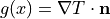. The integral term over the Neumann surface in the equation (4) contains exactly the same flux. So we can use the known function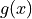in the integral term: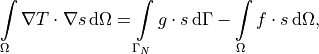where test functionalso belongs to the space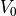.

That is why Neumann conditions in FEM terminology are called Natural Boundary Conditions. These conditions are a part of weak form terms.

## The weak form of the Poisson’s equation¶

Now we can write the resulting weak form for the Poisson’s problem (1)(3). For any test functionfindsuch that

(5)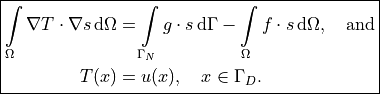## Discussion of discretization and meshing¶

It is planned to have an example of the discretization based on the Poisson’s equation weak form (5). For now, please refer to the wikipedia page Finite Element Method for a basic description of the disretization and meshing.

## Numerical solution of the problem¶

To solve numerically given problem based on the weak form (5) we have to go through 5 steps:

1. Define geometry of the domain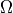and surfacesand.

2. Define the known functions,and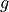.

3. Define the unknown functionand the test functions.

4. Define essential boundary conditions (Dirichlet conditions)5. Define equation and natural boundary conditions (Neumann conditions) as the set of all integral terms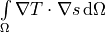,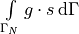,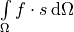.